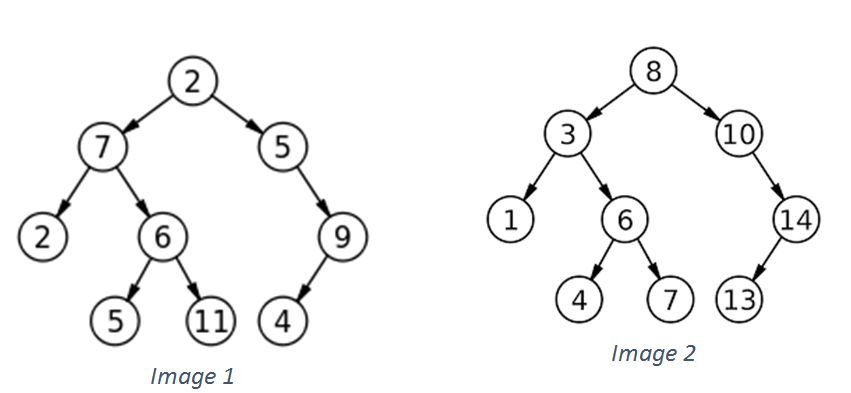# Find whether two trees are structurally identical or not | Data Structure

Given two binary trees, we need to check whether two trees are structurally identical or not.
Submitted by Radib Kar, on October 09, 2018

Solution:

Since we need to check only structural similarity we needn’t check their respective node values, rather we need to check whether both have the same structural organization or not. So the simple algorithm to solve it can be:

1. If both trees are NULL, then they are structurally similar.
2. If one of the two is NULL and other is not NULL, then they are not structurally similar.
3. If both of the trees are not NULL we recursively check right and left subtree.

Example:Fig: Two tree which are structurally identical but are not similar
Image source: wikipedia

Functional problem:

We need to write a Boolean function where we are given two roots for two trees.

```    bool IsSimilar(Tree* root1, Tree* root2);
```

Pseudocode:

```bool IsSimilar( Tree* root1, Tree* root2){
// both tree empty
if(root1==NULL && root2==NULL)
return true;
// one tree empty, another is not
if(root1==NULL || root2==NULL)
return false;

// above two are base conditions

//if both tree is not empty
// recursively check for left and right subtrees
return (IsSimilar(root1->left,root2->left) && IsSimilar(root1->right,root2->right));
}
```

C++ implementation:

```#include <bits/stdc++.h>
using namespace std;

// tree node is defined
class tree{
public:
int data;
tree *left;
tree *right;
};

bool IsSimilar( tree *root1, tree* root2){
// both tree empty
if(root1==NULL && root2==NULL)
return true;
// one tree empty, another is not
if(root1==NULL || root2==NULL)
return false;

// above two are base conditions

//if both tree is not empty
// recursively check for left and right subtrees
return (IsSimilar(root1->left,root2->left) && IsSimilar(root1->right,root2->right));
}

// creating new node
tree* newnode(int data)
{
tree* node = (tree*)malloc(sizeof(tree));
node->data = data;
node->left = NULL;
node->right = NULL;

return(node);
}

int main()
{
//**same trees are built as shown in example**

// building first tree
tree *root1=newnode(2);
root1->left= newnode(7);
root1->right= newnode(5);
root1->right->right=newnode(9);
root1->right->right->left=newnode(4);
root1->left->left=newnode(2);
root1->left->right=newnode(6);
root1->left->right->left=newnode(5);
root1->left->right->right=newnode(11);
cout<<"first tree is built,same as image1"<<endl;

// building second tree
tree *root2=newnode(8);
root2->left= newnode(3);
root2->right= newnode(10);
root2->right->right=newnode(14);
root2->right->right->left=newnode(13);
root2->left->left=newnode(1);
root2->left->right=newnode(6);
root2->left->right->left=newnode(4);
root2->left->right->right=newnode(7);

cout<<"second tree is built,same as image2"<<endl;
if(IsSimilar(root1,root2))
cout<<"Both are structurally similar"<<endl;
else
cout<<"Both aren't structurally similar"<<endl;

return 0;
}
```

Output

```first tree is built,same as image1
second tree is built,same as image2
Both are structurally similar
```

Let’s consider another thing:

If the question was whether two trees are identical or not then we had to check the node data’s also. A little bit modification like below would have been enough.

Modified pseudocode:

```bool IsSimilar( Tree* root1, Tree* root2){
// both tree empty
if(root1==NULL && root2==NULL)
return true;
// one tree empty, another is not
if(root1==NULL || root2==NULL)
return false;

// above two are base conditions

//if both tree is not empty
// recursively check for left and right subtrees
return ( (root1->data==root2->data) && IsSimilar(root1->left,root2->left) && IsSimilar(root1->right,root2->right));
// check the modification for comapring node' data also
}
```